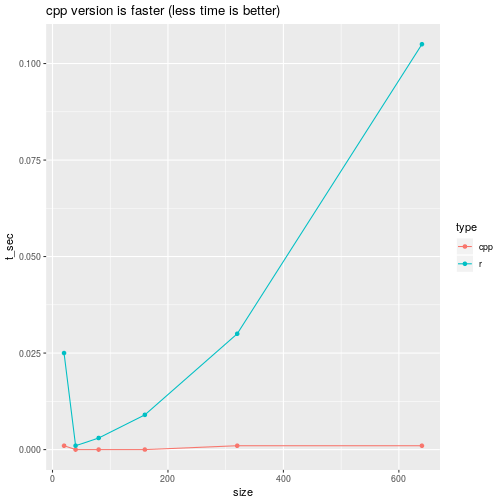Goal if this vignette is to compare the run-time speeds of `calc_act` (the C++ version) and `calc_act_r` (the R version).

``````library(tracerer)
``````

Create a trace:

``````trace <- sin(seq(from = 0.0, to = 2.0 * pi, length.out = 10))
``````

Every size, the size of `trace` is doubled.

``````n_sizes <- 6
n_types <- 2
elapseds <- data.frame(
type = as.factor(rep(c("cpp", "r"), times = n_sizes)),
size = rep(NA, times = n_sizes * n_types),
t_sec = rep(NA, times = n_sizes * n_types)
)

for (i in seq(1, n_sizes))
{
# Duplicate input
trace <- c(trace, trace)

# Measure again
t_r <- rbenchmark::benchmark(
tracerer::calc_act_r(trace, sample_interval = 2),
replications = 1,
columns = c("elapsed")
)\$elapsed
t_cpp <- rbenchmark::benchmark(
tracerer::calc_act(trace, sample_interval = 2),
replications = 1,
columns = c("elapsed")
)\$elapsed

elapseds\$size[(i * 2) - 1] <- length(trace)
elapseds\$size[i * 2]  <- length(trace)
elapseds\$t_sec[(i * 2) - 1] <- t_cpp
elapseds\$t_sec[i * 2] <- t_r
}
``````

In a plot:

``````ggplot2::ggplot(
data = elapseds,
ggplot2::aes(x = size, y = t_sec, color = type)
) + ggplot2::geom_line() +
ggplot2::geom_point() +
ggplot2::ggtitle("cpp version is faster (less time is better)")
``````